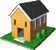Ontario Research Centre for Computer Algebra Technical Reports
The Ontario Research Centre for Computer Algebra

# The UWO ORCCA Reading Room

## UWO ORCCA TR-05-03 Summary

Quasipolynomial rootfinding, a numerical homotopy method, by Ashley B. Pitcher and Robert M. Corless. This paper was presented at the Canadian Undergraduate Mathematics Conference in Queen's University, 2005.

Abstract: Quasipolynomials arise in a number of applications, and in particular as the characteristic equation associated with systems of delay differential equations. Quasipolynomial equations are generally difficult to solve, and most often must be solved numerically. In the non-trivial case, quasipolynomials have an infinite number of roots in the complex plane. We present a new numerical method for finding the roots of any given quasipolynomial that lie in a given region of the complex plane. The method is based on homotopy continuation, a method currently used to solve polynomial systems. A homotopy between two functions $Q$ and $P$ from a space $X$ to a space $Y$ is a continuous map $H(\lambda,\mu)$ from $X \times [0,1] \mapsto Y$ such that $H(\lambda,0) = Q(\lambda)$ and $H(\lambda,1) = P(\lambda)$. In our case space $X$ and space $Y$ are both the complex plane and $\mu$ is a parameter that varies from $0$ to $1$. $Q(\lambda)$ represents a problem that is easier to solve (the roots are known or can be easily computed). $P(\lambda)$ is the original quasipolynomial. We begin with the roots of $Q(\lambda)$ (which are the roots of $H$ at $\mu = 0$). As $\mu$ increases from $0$ to $1$, numerical continuation methods trace out the homotopy paths. At $\mu = 1$, the roots of $H$ are also the roots of $P(\lambda)$, the original quasipolynomial. The numerical continuation method used to trace the homotopy paths will be discussed as well as our future work in this area.

 About ORCCA Members Events & SeminarsORCCA Home Research Activities Positions Reading Room Contact Info